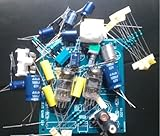# Nobsound® 6J1 tube Pre-AMP Preamplifier DIY KITParameters:

Filament voltage (Uf) = 6.3V;
Filament current (If) = 0.17A;
Anode voltage (Ua) = 120V;
Anode current (Ia) 4.5 ~ 12mA;
The second gate voltage (Ug2) = 120V;
The second gate current (Ig2) ≤3.2mA;
Transconductance (S) = 5.2 ± 1.4mA / V.
(Electrode capacitance) input capacitance (Cin) = 4.3pF;
Output capacitance (Cout) = 2.35pF;
Transition capacitance (Cag) ≤0.02pF;
Between the filament and the cathode capacitance (Cfk) = 4.65pF
(Limit the use of data) maximum filament voltage (Ufmax) = 7V;
The minimum filament voltage (Ufmin) = 5.7V;
Maximum anode voltage (Uamax) = 200V;
The second largest gate voltage (Ug2maxp) = 150V;
The maximum voltage between the filament and the cathode (Ufkmax) ±

Sale Price:\$30.00

[amazon-product-reviews]